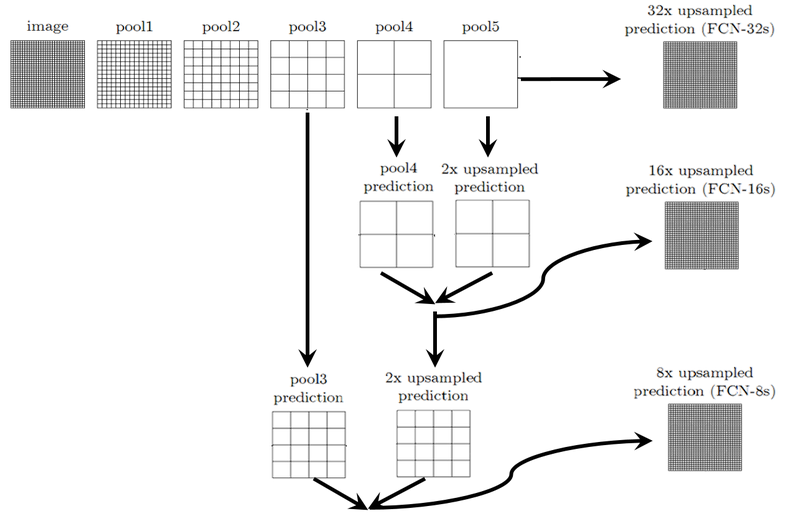• 使用python语言和pytorch框架简单的复现FCN模型，数据集为100个书包的图片，并使用FCN模型对其进行分类。
• ## FCN

千次阅读 2018-04-15 23:16:05
来源：https://blog.csdn.net/zhyj3038/article/details/711952621 实例分割,对图像中的每一个像素点进行分类,同种物体的不同实例也用不同的类标进行标注，中间是实例分割,右图是语义分割.2FCN与CNN区别3CNN中...
来源：https://blog.csdn.net/zhyj3038/article/details/71195262
1 实例分割,
对图像中的每一个像素点进行分类,同种物体的不同实例也用不同的类标进行标注，中间是实例分割,右图是语义分割.2FCN与CNN区别

def AlexNet(images, classNum=None, dropoutrate=None):
parameters = []

# 卷积层1
conv1, parameters = convLayer(images, name='conv1', kh=11, kw=11, n_out=64, dh=4, dw=4, p=parameters)
# 添加LRN层和最大池化层
# 对conv1进行LRN处理
lrn1 = tf.nn.lrn(conv1, 4, bias=1.0, alpha=0.001/9, beta=0.75, name='lrn1')
pool1 = tf.nn.max_pool(lrn1, ksize=[1, 3, 3, 1], strides=[1, 2, 2, 1], padding='VALID', name='pool1')
# 打印出结果pool1的结构
print pool1.op.name, ' ', pool1.get_shape().as_list()

# 卷积层2
conv2, parameters = convLayer(pool1, name='conv2', kh=5, kw=5, n_out=192, dh=1, dw=1, p=parameters)
# LRN处理
lrn2 = tf.nn.lrn(conv2, 4, bias=1.0,alpha=0.001/9, beta=0.75, name='lrn2')
# 最大池化处理
pool2 = tf.nn.max_pool(lrn2, ksize=[1, 3, 3, 1], strides=[1, 2, 2, 1], padding='VALID', name='pool2')
# 打印出结果pool2的结构
print pool2.op.name, ' ', pool2.get_shape().as_list()

# 卷积层3
conv3, parameters = convLayer(pool2, name='conv3', kh=3, kw=3, n_out=384, dh=1, dw=1, p=parameters)

# 卷积层4
conv4, parameters = convLayer(conv3, name='conv4', kh=3, kw=3, n_out=256, dh=1, dw=1, p=parameters)

# 卷积层5
conv5, parameters = convLayer(conv4, name='conv5', kh=3, kw=3, n_out=256, dh=1, dw=1, p=parameters)
pool5 = tf.nn.max_pool(conv5, ksize=[1, 3, 3, 1], strides=[1, 2, 2, 1], padding='VALID', name='pool5')
print pool5.op.name, ' ', pool5.get_shape().as_list()

fc_in = tf.reshape(pool5, [-1, 256*6*6])
fc6 = fcLayer(fc_in, 256*6*6, 4096, True, 'fc6')
dropout6 = tf.nn.dropout(fc6, dropoutrate)

fc7 = fcLayer(dropout6, 4096, 4096,True, 'fc7')
dropout7 = tf.nn.dropout(fc7, dropoutrate)

fc8 = fcLayer(dropout7, 4096, classNum, True, 'fc8')

return pool5, parameters

FCN网络结构的部分代码，将三个全连接换成了卷积层：

with tf.variable_scope("inference"):
image_net = vgg_net(weights, processed_image)
conv_final_layer = image_net["conv5_3"]

pool5 = utils.max_pool_2x2(conv_final_layer)

W6 = utils.weight_variable([7, 7, 512, 4096], name="W6")
b6 = utils.bias_variable(, name="b6")
conv6 = utils.conv2d_basic(pool5, W6, b6)
relu6 = tf.nn.relu(conv6, name="relu6")
if FLAGS.debug:
#根据给出的keep_prob参数，将输入tensor x按比例输出。
relu_dropout6 = tf.nn.dropout(relu6, keep_prob=keep_prob)

W7 = utils.weight_variable([1, 1, 4096, 4096], name="W7")#图片的尺寸变成1*1，卷积核的数量4096，输出的尺寸就是1*1*4096，最后额
b7 = utils.bias_variable(, name="b7")
conv7 = utils.conv2d_basic(relu_dropout6, W7, b7)
relu7 = tf.nn.relu(conv7, name="relu7")
if FLAGS.debug:
relu_dropout7 = tf.nn.dropout(relu7, keep_prob=keep_prob)

W8 = utils.weight_variable([1, 1, 4096, NUM_OF_CLASSESS], name="W8")
b8 = utils.bias_variable([NUM_OF_CLASSESS], name="b8")
conv8 = utils.conv2d_basic(relu_dropout7, W8, b8)
# annotation_pred1 = tf.argmax(conv8, dimension=3, name="prediction1")

# now to upscale to actual image size 现在升级为实际的图像大小
deconv_shape1 = image_net["pool4"].get_shape()
W_t1 = utils.weight_variable([4, 4, deconv_shape1.value, NUM_OF_CLASSESS], name="W_t1")
b_t1 = utils.bias_variable([deconv_shape1.value], name="b_t1")
conv_t1 = utils.conv2d_transpose_strided(conv8, W_t1, b_t1, output_shape=tf.shape(image_net["pool4"]))

deconv_shape2 = image_net["pool3"].get_shape()
W_t2 = utils.weight_variable([4, 4, deconv_shape2.value, deconv_shape1.value], name="W_t2")
b_t2 = utils.bias_variable([deconv_shape2.value], name="b_t2")
conv_t2 = utils.conv2d_transpose_strided(fuse_1, W_t2, b_t2, output_shape=tf.shape(image_net["pool3"]))

shape = tf.shape(image)
deconv_shape3 = tf.stack([shape, shape, shape, NUM_OF_CLASSESS])
W_t3 = utils.weight_variable([16, 16, NUM_OF_CLASSESS, deconv_shape2.value], name="W_t3")
b_t3 = utils.bias_variable([NUM_OF_CLASSESS], name="b_t3")
conv_t3 = utils.conv2d_transpose_strided(fuse_2, W_t3, b_t3, output_shape=deconv_shape3, stride=8)

annotation_pred = tf.argmax(conv_t3, dimension=3, name="prediction")

return tf.expand_dims(annotation_pred, dim=3), conv_t3
#对conv8此时进行32倍的上采样可以得到原图大小,这个时候得到的结果就是叫做FCN-32s.
#在FCN-32s的基础上进行fine tuning,把pool4层和conv8的2倍上采样结果相加之后进行一个16倍的上采样,得到的结果是FCN-16s.如conv_t1
#FCN-16s的基础上进行fine tuning,把pool3层和2倍上采样的pool4层和4倍上采样的conv8层加起来,进行一个8倍的上采样,得到的结果就是FCN-8s

4 FCN的优点和缺点：
FCN的优点,能够end-to-end, pixels-to-pixels,而且相比于传统的基于cnn做分割的网络更加高效,因为避免了由于使用像素块而带来的重复存储和计算卷积的问题。
FCN的缺点也很明显,首先是训练比较麻烦,需要训练三次才能够得到FCN-8s,而且得到的结果还是不精细,对图像的细节不够敏感,这是因为在进行decode,也就是恢复原图像大小的过程时,输入上采样层的label map太稀疏,而且上采样过程就是一个简单的deconvolution.
其次是对各个像素进行分类,没有考虑到像素之间的关系.忽略了在通常的基于像素分类的分割方法中使用的空间规整步骤,缺乏空间一致性.
5 FCN的实施过程中的跳跃连接的理解：
这个结构的作用就在于优化结果，因为如果将全卷积之后的结果直接上采样得到的结果是很粗糙的，所以作者将不同池化层的结果进行上采样之后来优化输出。具体结构如下：6 U-net：
和FCN相比,结构上比较大的改动在上采样阶段,上采样层也包括了很多层的特征.
还有一个比FCN好的地方在于,Unet只需要一次训练,FCN需要三次训练.


展开全文• fcn_score 计算fcn分数以进行GAN评估 用法： 首次运行download_fcn.sh python Evaluation.py --result_dir = <具有结果图像的目录> --gt_dir = <具有基础图像的目录> --gpu_id = 0 --output_dir = result /Python
• 使用FCN的密集网的张量流实现以进行肝脏分割。 逐步配置 模型的所有基本配置和训练过程均在config.py中列出，并由EasyDict管理。 我们将配置参数定义为__C ，该参数在config.py定义。 注意：所有配置都可能会在一...Python
• FCN实现手写数字数据集MNIST的识别，代码为自己翻写，包含MNIST数据集，代码与大量注释，及24页原创报告
• fcn内网穿透MAC版，可局域网穿透使用虚拟网络对服务器运维
• MALSTM-FCN_TensorflowKeras2 MALSTM-FCN基本实现 这是MALSTM-FCN的Tensorflow2实现，用于时间序列分类。 可在作者的存储库网页上找到与Tensorflow1和Keras兼容的原始存储库： : 请参考上面的网页以获取有关模型...Python
• FCN网络实现（pytorch），需要torchvison的VGG预训练模型，输出经过nn.logsoftmax处理后，使用nn.NLLLoss()损失函数。
• pytorch_fcn FCN的pytorch实施（VGG，ResNet）
• MLSTM FCN模型（来自 FCN）使用最新的单变量时间序列模型（来自的最新的单变量时间序列模型，LSTM-FCN和ALSTM-FCN来增强挤压和激励块。 对于LSTM-FCN和ALSTM-FCN模型的代码可以在这里找到 。 安装 下载存储库并应用...
• fcn模型的搭建代码，可以根据这个代码实现fcn的搭建以及训练，测试
• FCN模型的网络与VGG16类似，之后后边将全连接层换成了卷基层，具体的网络结构与细节可以去看论文： https://people.eecs.berkeley.edu/~jonlong/long_shelhamer_fcn.pdf 下边详细讲一下用Pytorch对FCN的实现： 本文...
• 实施不是原始文件。 该项目的目的是开始语义分割并掌握基本过程。 发布FCN32 / 8，SegNet，U-Net谢谢您的支持。 环境 物品 价值 物品 价值 ...FCN32 可视化结果： FCN8 可视化结果： 隔离网 网络
• torchvison的fcn_resnet50_coco-1167a1af图像分割预训练模型，里面包含fcn_resnet50和resnet50的预训练模型，二者缺一不可。如果使用torchvision自动下载比较慢的可以直接下载这个放在%USERPROFILE%.cache\torch\hub...
• 基于PASCAL的FCN网络结构图，使用Caffe深度学习框架绘制，内容为FCN8s.png，FCN16s.png，FCN32s.png
• 包含fcn8s-heavy-pascal.caffemodel、fcn8s-heavy-pascal.prototxt、pascal-classes.txt文件 和FCN模型实现图像分割源码、配套图片素材 具体实现效果见CSDN博客“DNN系列5_FCN模型实现图像分割”查看效果
• pytorch-fcn PyTorch实现。 要求 > = 0.2.0 > = 0.1.8 > = 6.1.5 安装 git clone https://github.com/wkentaro/pytorch-fcn.git cd pytorch-fcn pip install . # or pip install torchfcn 训练 参见。 准确性 在...
• 多分类，FCN代码，cuda8.0、cudnn5.1、TensorFlow1.4.0,资源包含了对github代码的修改
• python所写的语义分割代码，采用Pytorch框架，代码完整，完美运行。
• 用于对象检测和分割的Mask R-CNN +用于语义分割的FCN 该软件伴随着我的论文项目“在深度卷积神经网络中使用上下文提示进行对象检测”期间进行的工作。 所提出的架构是一个两阶段的实现，包括对Python 3，Keras和...
• FCN-for-Semantic-Segmentation 实现和测试 FCN-16 和 FCN-8 的性能。 除此之外，CRF 被用作后处理技术并比较结果。 参考文献： 用于语义分割的全卷积网络 作者：乔纳森·朗、埃文·谢尔哈默、特雷弗·达雷尔 链接：...
• 解压之后的文件改名为.gz后缀再解压，里面的文件分别是resnet50_rfcn_final.caffemodel和resnet101_rfcn_final.caffemodel
• 使用python语言和pytorch框架简单的复现FCN模型
• UNet / FCN PyTorch 该存储库包含U-Net和FCN的简单PyTorch实现，这是Ronneberger等人提出的深度学习细分方法。 和龙等。 用于训练的合成图像/遮罩 首先克隆存储库并cd到项目目录。 import matplotlib . pyplot as ...
• 图像分割Keras：在Keras中实现Segnet，FCN，UNet，PSPNet和其他模型。 在Keras中实现各种深度图像分割模型。 链接到包含教程的完整博客文章： : 有效的Google Colab示例： Python介面： : CLI界面： ： 我们的...Python
• 自己精心整理的"FCN 实现图像语义分割"的视频讲解,mp4,华文讲解,很详细!
• FCN是一款无需公网IP即可实现远程接入局域网的软件，傻瓜式的接入代理方便小巧
• 此文件描述了FCN 代码工程的运行步骤，讲述了所需要的配置环境，和每一步运行后的结果，最终给出了训练模型结束后的分割结果。
• 3D-2D-FCN 是在“ caffe”框架上实现的； 因此，您的系统需要“ caffe”框架作为运行此项目的先决条件。 您可以按照以下步骤操作： 首先，从（仅30张火车图像及其标签）下载腹部CT扫描。 这些文件具有.nii扩展名...
• SpixelFCN：具有完全卷积网络的超像素分割 这是CVPR-20文件中介绍的超像素分割网络的PyTorch实施： ，，和 如有任何疑问，请联系（ ）。 先决条件 该培训代码主要是使用python 2.7，PyTorch 0.4.1，CUDA 9和Ubuntu ...Python...